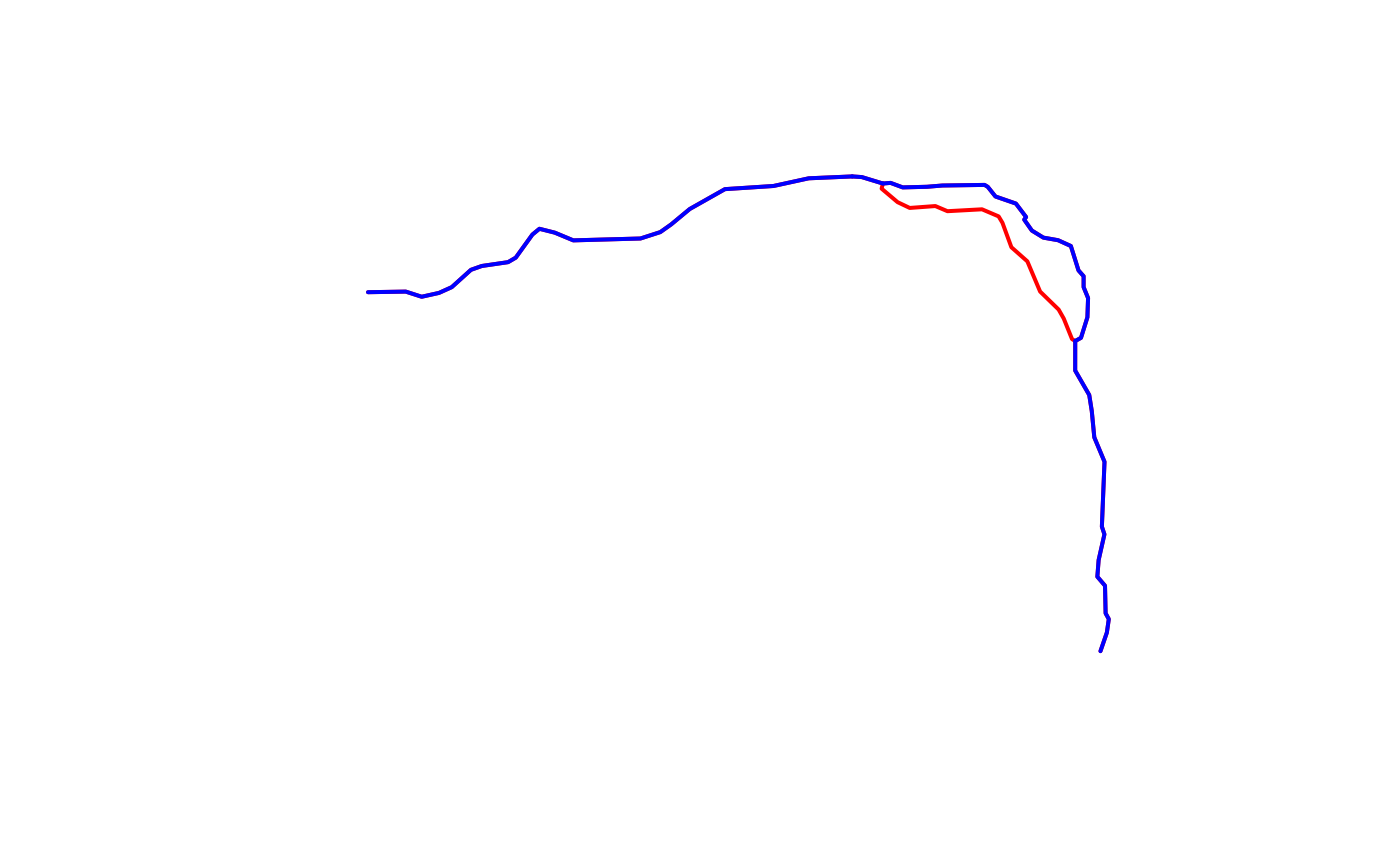Traverse NHDPlus network downstream with diversions NOTE: This algorithm may not scale well in large watersheds. For reference, the lower Mississippi will take over a minute.

get_DD(network, comid, distance = NULL)

## Arguments

network

data.frame NHDPlus flowlines including at a minimum: COMID, DnMinorHyd, DnHydroseq, and Hydroseq.

comid

integer identifier to start navigating from.

distance

numeric distance in km to limit how many COMIDs are returned. The COMID that exceeds the distance specified is returned. The longest of the diverted paths is used for limiting distance.

## Value

integer vector of all COMIDs downstream of the starting COMID

## Examples

library(sf)
#> Linking to GEOS 3.11.2, GDAL 3.6.2, PROJ 9.2.0; sf_use_s2() is TRUE
start_COMID <- 11688818

source(system.file("extdata", "sample_flines.R", package = "nhdplusTools"))

DD_COMIDs <- get_DD(sample_flines, start_COMID, distance = 4)
plot(dplyr::filter(sample_flines, COMID %in% DD_COMIDs)$geom, col = "red", lwd = 2) DM_COMIDs <- get_DM(sample_flines, start_COMID, distance = 4) plot(dplyr::filter(sample_flines, COMID %in% DM_COMIDs)$geom,
col = "blue", add = TRUE, lwd = 2)